Next: Corrected Implementation Up: Incorrect Implementation Previous: Incorrect Implementation

## PVS analysis

Although we knew the flaw in this protocol before beginning the analysis, we proceeded with a mechanical analysis, to see where it broke down, and whether we could make any deductions from that.

In fact, the flaw revealed itself very quickly. The new generates function, which includes XOR, is given by:

  Gen(S)(m) : INDUCTIVE bool =
S(m)
OR  (EXISTS m1, m2 : Gen(S)(m1) AND Gen(S)(m2) AND m = conc(m1, m2))
OR  (EXISTS m1 : Gen(S)(conc(m1, m)))
OR  (EXISTS m2 : Gen(S)(conc(m, m2)))
OR  (EXISTS m1 : Gen(S)(m1) AND m = hash(m1))
OR  (EXISTS m1, m2 : Gen(S)(m1) AND Gen(S)(m2) AND m = xor(m1, m2))
OR  (EXISTS m1 : Gen(S)(m1) AND Gen(S)(xor(m1, m)))
OR  (EXISTS m2 : Gen(S)(m2) AND Gen(S)(xor(m, m2)));


It is impossible to prove that the blank'' rank function

 rho(m) : RECURSIVE int =
CASES m OF
text(z)     : 1,
nonce(z)    : 1,
user(z)     : 1,
session(z)  : IF session(z) = session(sk(a, b)) THEN 0 ELSE 1 ENDIF,
longterm(z) : IF z = lt(a) OR z = lt(b) THEN 0 ELSE 1 ENDIF,
conc(z1, z2): min(rho(z1), rho(z2)),
hash(z1)    : 1,
xor(z1, z2) : 1
ENDCASES
MEASURE size(m)


is valid, in other words an attempted proof of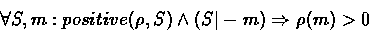fails. It requires a sublemma: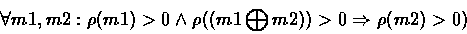and the counter-example is that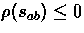, since it is secret, but the enemy may know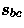, since he may be masquerading as agent C , and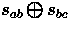is also known, since it circulates in the network, so the proof of the sublemma fails.

Other rank functions could be tried, in which case the proof would fail at some other stage.